# How to write Ohm symbol in LaTeX like Ω?

Ohm(Ω) symbol is represented by the Greek capital letter Omega. This greek letter omega is represented in the document by \Omega and \textOmega commands.

However, this \textOmega command does not default, for this you need to take the help of \testgreek package.

\documentclass{article}
\usepackage{textgreek}
\begin{document}
100\Omega - 40\Omega = 60\textOmega
\end{document}

Output :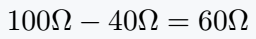You can define your own commands by modifying Omega. You can see by looking at this LaTeX program below that the value of resistance has been passed as an argument with the new command.

And the symbol will move automatically with the value.

\documentclass{article}
\newcommand\Ohm{#1\Omega}
\begin{document}
$r_1 = \Ohm{20}$
$r_2 = \Ohm{30}$
$r_1 + r_2 = \Ohm{50}$
\end{document}

Output :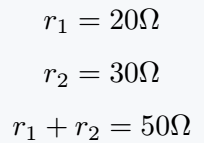## Use different packages for Ohm symbol

Different commands are written in different external packages just for this Ω symbol. For example

### 1. Mathcomp package for \tcohm command

You can use \tcohm only in math mode.

\documentclass{article}
\usepackage{mathcomp}
\begin{document}
1M$\tcohm = 10^6 \tcohm$ \$6pt] 1K\tcohm = 10^3 \tcohm \\[6pt] 1m\tcohm = 10^{-3} \tcohm \end{document} Output :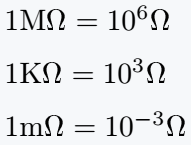### 2. Textcomp package for \textohm command As you can see from the package and command name, this \textohm is only valid for text mode. \documentclass{article} \usepackage{textcomp} \begin{document} 1G\textohm =  10^9 \textohm \\[6pt] 1M\textohm =  10^6 \textohm \\[6pt] 1K\textohm =  10^3 \textohm \end{document} Output :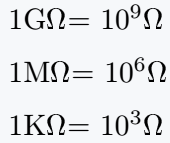### 3. Gensymb package for \ohm command In my opinion, the best practice is to use \ohm command for the Ω symbol over the other two commands, and you can use this command in both math and text mode. \documentclass{article} \usepackage{gensymb} \begin{document} 1n\ohm =  10^{-9} \ohm  \\ [6pt] 1m\ohm =  10^{-3} \ohm  \\ [6pt] 1p\ohm =  10^{12} \ohm  \end{document} Output :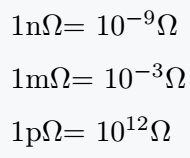## Use siunitx package for Ω symbol Whenever you define a unit symbol with the help of latex, you must use the siunitx package. Because this package has been specially imported in Latex for the solution of unit-related problems. \documentclass{article} \usepackage{siunitx} \begin{document} \[ R_a=\qty{20}{\ohm}$
$R_b=\qty{70}{\ohm}$
$R_c=\qty{90}{\ohm}$
\end{document}

Output :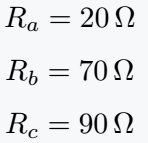Best practice in LaTeX version3 is to use \qty command instead of SI and si.

In one of these, the value is used as an argument and in other, the \ohm command is used. As a result, value ​​and unit are printed together in pdf.

You can do different styles by passing color and unit color properties as an optional argument in \qty command.

\documentclass{article}
\usepackage{siunitx}
\begin{document}
$R_a=\qty[color=blue]{20}{\ohm}$
$R_b=\qty[unit-color=red]{70}{\ohm}$
$R_c=\qty[color=magenta]{90}{\ohm}$
\end{document}

Output :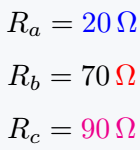color properties will convert both value and unit together to blue and magenta color. And, unit-color properties will only convert Unit to red color.

Direct commands for mega, kilo, and milliohms are present in this siunitx.

\documentclass{article}
\usepackage{siunitx}
\begin{document}
$R_a=\qty[unit-color=blue]{20}{\Mohm}$
$R_b=\qty[unit-color=red]{70}{\kohm}$
$R_c=\qty[unit-color=magenta]{90}{\mohm}$
\end{document}

Output :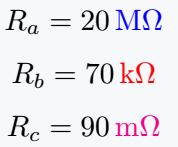#### Md Jidan Mondal

LaTeX expert with over 10 years of experience in document preparation and typesetting. Specializes in creating professional documents, reports, and presentations using LaTeX.